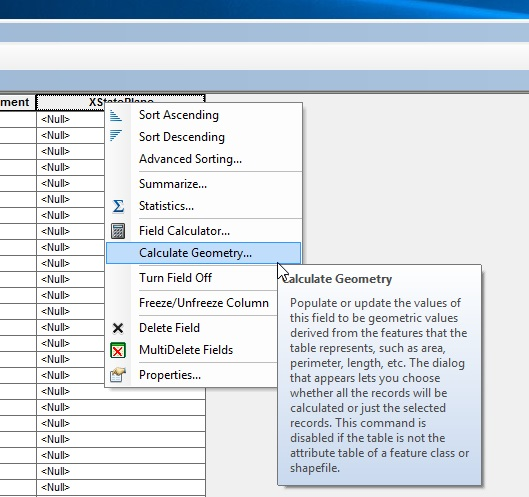# Converting Lat/Long to State Plane and populating Easting/Northing in table

4674
2
09-26-2017 05:22 AMNew Contributor II

I have a fairly large table with Lat/Long coordinates, brought over from a field collector. Customer wants a shapefile in state plane coords (which is not a problem), and also wants those state plane coords to show in the existing table. What is the best way to convert and populate the Easting/Northing fields from the existing Lat/Long fields in the table?

Help will be greatly appreciated.

Tags (2)
1 Solution

Accepted SolutionsbyMVP Frequent Contributor

If the data is brought into GIS as a feature class, one can create fields and populate the coordinates for the coordinate system of your choice using Calculate Geometry

The Calculate Geometry tool allows you to access the geometry of the features in a layer. The tool can calculate coordinate values, lengths, and areas, depending on the geometry of the input layer. You can only calculate the area, length, or perimeter of features if the coordinate system being used is projected. Keep in mind that different projections have different spatial properties and distortions. If the coordinate system of the data source and data frame are not the same, you may get a different result if you calculate geometry using the data frame's coordinate system than when you calculate using the data source's coordinate system.Alternately, one could use XTools Pro, a third-party software that fits into ArcGIS Desktop.  It has an "Add XYZ Coordinates" function (under Table Operations) that works in a similar manner, but does both X and Y at the same time.

https://xtools.pro/en/overview/

Chris Donohue, GIS

2 RepliesbyMVP Frequent Contributor

If the data is brought into GIS as a feature class, one can create fields and populate the coordinates for the coordinate system of your choice using Calculate Geometry

The Calculate Geometry tool allows you to access the geometry of the features in a layer. The tool can calculate coordinate values, lengths, and areas, depending on the geometry of the input layer. You can only calculate the area, length, or perimeter of features if the coordinate system being used is projected. Keep in mind that different projections have different spatial properties and distortions. If the coordinate system of the data source and data frame are not the same, you may get a different result if you calculate geometry using the data frame's coordinate system than when you calculate using the data source's coordinate system.Alternately, one could use XTools Pro, a third-party software that fits into ArcGIS Desktop.  It has an "Add XYZ Coordinates" function (under Table Operations) that works in a similar manner, but does both X and Y at the same time.

https://xtools.pro/en/overview/

Chris Donohue, GISNew Contributor II

Yes. Thank you so much. I found and remembered that just moments ago. Had a brain lapse, I guess.

Thank you again very much for your help.